# Twin-T band-pass filter puzzle

#### PeteHL

Joined Dec 17, 2014
340
In the schematic, the configuration A, inverting active band-pass filter, functions as expected with voltage gain at the resonant frequency of 5 kHz that approaches 10. This is from a book about op amps.

Configuration B is an attempt to do the same thing as A except in a non-inverting amplifier configuration so that voltage gain is never less than 1 (unity). For this config., voltage gain at 5 kHz is greater than 100.

Given that

(Ri + Rf) / Ri = 11

how is it possible for voltage gain of Config. B to be > 100?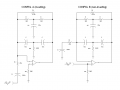#### crutschow

Joined Mar 14, 2008
24,911
how is it possible for voltage gain of Config. B to be > 100?
Don't see how it can be above 11.#### PeteHL

Joined Dec 17, 2014
340
Don't see how it can be above 11.Tested Config. B simulated and at my workbench, in both cases voltage gain "through the roof" at 5 kHz.

Maybe I will check again. But if inclined, please try computer simulation, such a simple circuit builds quickly.

-Pete

#### Tesla23

Joined May 10, 2009
403
Tested Config. B simulated and at my workbench, in both cases voltage gain "through the roof" at 5 kHz.

Maybe I will check again. But if inclined, please try computer simulation, such a simple circuit builds quickly.

-Pete
The configurations are very different, config B is sensitive to the loading by the twin-T network on the inverting input whereas config A isn't. My rough estimate of the gain of config B at 5kHz is about 200.

Imaging adding a 1k resistor from the inverting input to ground in each configuration. In A there would be no change, in B the gain would increase. This is what is happening, the Twin-T network loads the inverting input with about 2.3k at 5kHz in both A and B, in A it has no effect on the gain, in B it increases it dramatically.

#### PeteHL

Joined Dec 17, 2014
340
The configurations are very different, config B is sensitive to the loading by the twin-T network on the inverting input whereas config A isn't. My rough estimate of the gain of config B at 5kHz is about 200.

Imaging adding a 1k resistor from the inverting input to ground in each configuration. In A there would be no change, in B the gain would increase. This is what is happening, the Twin-T network loads the inverting input with about 2.3k at 5kHz in both A and B, in A it has no effect on the gain, in B it increases it dramatically.
Except that at resonance, in theory at least, current through each T is equal and of opposite phase and so it is as if the twin T is not in the circuit at all. Or in other words, impedance of the twin T is very high. Based on the operation of Config. A, at resonance, Impedance of the twin T must be close to five times Rf.

#### Tesla23

Joined May 10, 2009
403
Except that at resonance, in theory at least, current through each T is equal and of opposite phase and so it is as if the twin T is not in the circuit at all. Or in other words, impedance of the twin T is very high. Based on the operation of Config. A, at resonance, Impedance of the twin T must be close to five times Rf.
The twin-T does not go completely high impedance at the notch frequency, the input and output still have a finite impedance to ground - and this is what explains the behaviour.

The Twin-T is a 3-terminal network: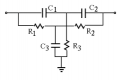and can be represented as a star network: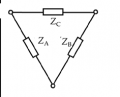and at the notch frequency, Zc becomes infinite - hence zero transmission, but Za and Zb are still far from infinite. Zc is in parallel with Rf in your circuit, so at the notch frequency when it goes o/c, the only feedback is Rf. However, Za is still in parallel with Ri and for Ri = 47k, dominates the gain. I estimate |Za| at the notch frequency to be R/sqrt(2) or about 2.3k. This explains the high gain.

One way to analyse the Twin-T is as two star networks in parallel, and do a star-delta transformation on each to get the equivalent star network.

Yes, it's the phase-shift of the Twin-T that I didn't account for.
In essence, it makes the feedback positive instead of negative at the resonant frequency, causing the high peak in the gain
At the resonant point, the Twin-T Impedance becomes very low, not high, as can be seen from the plot of the op amp output current.
I think that Zc from the Twin-T goes open circuit at the notch frequency, so the feedback doesn't become positive (is simply Rf), but because Za loads the inverting input the gain is so high, that the current you are seeing is simply the resultant high output voltage driving Zb.

#### PeteHL

Joined Dec 17, 2014
340
Crutschow and Tesla23,

Thanks very much for your explanations. Regretfully not being an engineer I only partially follow what you are saying. With some study I'll get a better grasp of it.

Melen and Garland, the engineers who came up with configuration A, must have known what you are explaining.

Thanks for exploring this,
Pete

#### The Electrician

Joined Oct 9, 2007
2,778
I get this response plot for Configuration A, as did others: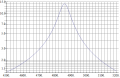For Configuration B, I get this response, again as have others: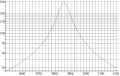To get what you said you wanted in the non-inverting configuration, you need to disconnect the center R and C which go to ground from the middle of the Twin T, and connect them to the non-inverting input of the opamp so that they will be driven by the input signal. Then you will get this response: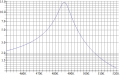#### Tesla23

Joined May 10, 2009
403
To get what you said you wanted in the non-inverting configuration, you need to disconnect the center R and C which go to ground from the middle of the Twin T, and connect them to the non-inverting input of the opamp so that they will be driven by the input signal.
Neat trick!

I'd suggest an improvement would be to drive the old RC ground with a unity gain buffer from the non-inverting input. Otherwise I think the input impedance has a negative real part due to the positive feedback.

#### The Electrician

Joined Oct 9, 2007
2,778
Neat trick!

I'd suggest an improvement would be to drive the old RC ground with a unity gain buffer from the non-inverting input. Otherwise I think the input impedance has a negative real part due to the positive feedback.
Of course all these analyses have assumed that the signal source driving the filter is an ideal voltage source with zero impedance, in which case it doesn't matter what the input impedance of the filter is. Not only does the real part of the input impedance become negative a little above 1 kHz, but the magnitude of the input impedance is very small around 5 kHz. Also, the behavior of the input impedance is quite sensitive to the gain characteristic of the opamp. I assumed it was ideal in my plots, and I think everybody else did too.

I was motivated to lift the Twin-T grounds by this old paper: http://documents.tips/documents/input-and-ground-as-complements-in-active-filters.html